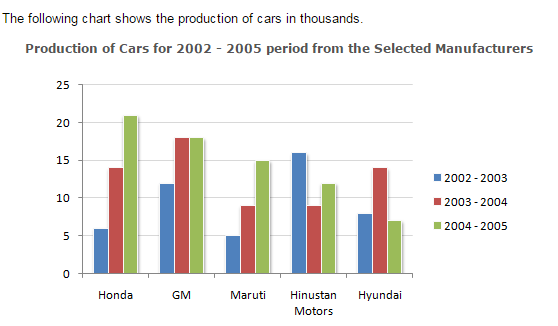Direction1.

How many companies have shown production below their average production in 2002 - 2003, but have showed above the average production in 2003 - 2004 ?

 A. One B. Two C. Three D. Four
Explanation: Average sales of company: Honda = (6 + 14 + 21)/3 = 13.66 GM = (12 + 18 + 18)/3 = 16 Maruti = (5 + 9 + 15)/3 = 9.66 Hindustan Motors= (16 + 9 + 12)/3 = 12.33 Hyundai = (8 + 14 + 7)/3 = 9.66

2.

The ratio of Hindustan Motors production in 2003 - 2004 to Honda's production in 2002 - 2003 is ?

 A. 0.66 B. 1.5 C. 2 D. None of these